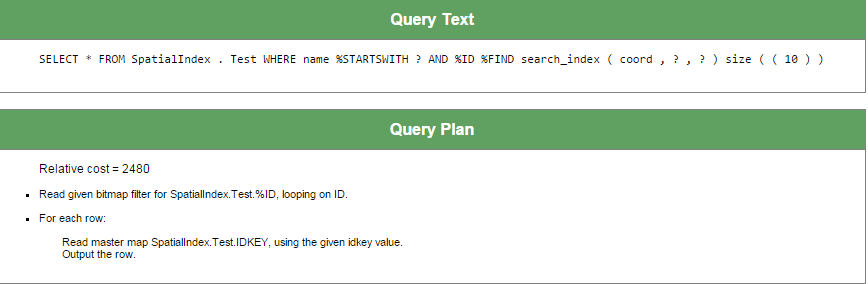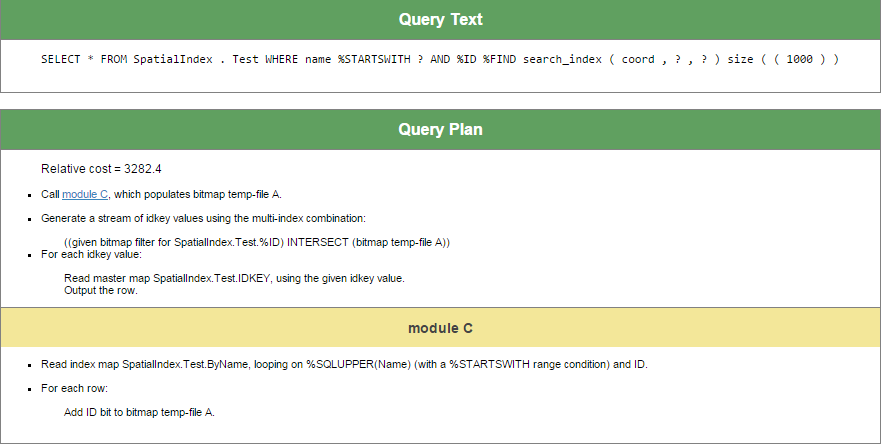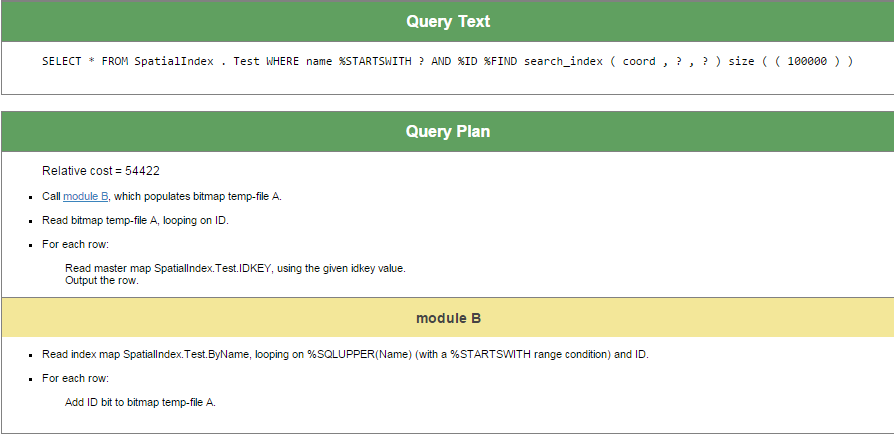﻿ Creating a Custom Index Type in Caché | InterSystems Developer Community |
Article
Alexander Koblov · Jan 29, 2016 9m read

# Creating a Custom Index Type in Caché

The object and relational data models of the Caché database support three types of indexes, which are standard, bitmap, and bitslice. In addition to these three native types, developers can declare their own custom types of indexes and use them in any classes since version 2013.1. For example, iFind text indexes use that mechanism.

A custom index type is a class that implements the methods of the %Library.FunctionalIndex interface to perform inserts, updates, and deletes. You can specify such a class as an index type when declaring a new index.

Example:

```Property A As %String;
Property B As %String;
Index someind On (A,B) As CustomPackage.CustomIndex;
```

The CustomPackage.CustomIndex class is the very class that implements custom indices.

For example, let's analyze the small prototype of a quadtree-based index for spatial data that was developed during the Hackathon by our team: Andrey Rechitsky, Aleksander Pogrebnikov, and me. (The Hackathon was organized at the annual InterSystems Russia Innovation School Training, and special thanks to the Hackathon's main inspiration, Timur Safin.)

In this article, I am not going to discuss quadtrees and how to work with them. Instead, let's examine how to create a new class which implements the %Library.FunctionalIndex interface for the existing quadtree algorithm implementation. In our team, this task was assigned to Andrey. Andrey created the SpatialIndex.Indexer class with two methods:

• Insert(x, y, id)
• Delete(x, y, id)

When creating a new instance of the SpatialIndex.Indexer class, it was necessary to define a global node name in which we store index data. All I needed to do was to create the SpatialIndex.Index class with the InsertIndex, UpdateIndex, DeleteIndex, and PurgeIndex methods. The first three methods accept the Id of the string to be modified and the indexed values exactly in the same order as they have been defined in the index declaration within the corresponding class. In our example, the input arguments are pArg(1)A and pArg(2)B.

```Class SpatialIndex.Index Extends %Library.FunctionalIndex [ System = 3 ]
{

ClassMethod InsertIndex(pID As %CacheString, pArg... As %Binary) [ CodeMode = generator, ServerOnly = 1 ]
{
if %mode'="method" {
set IndexGlobal = ..IndexLocation(%class,%property)
\$\$\$GENERATE(\$C(9)_"set indexer = ##class(SpatialIndex.Indexer).%New(\$Name("_IndexGlobal_"))")
\$\$\$GENERATE(\$C(9)_"do indexer.Insert(pArg(1),pArg(2),pID)")
}
}

ClassMethod UpdateIndex(pID As %CacheString, pArg... As %Binary) [ CodeMode = generator, ServerOnly = 1 ]
{
if %mode'="method" {
set IndexGlobal = ..IndexLocation(%class,%property)
\$\$\$GENERATE(\$C(9)_"set indexer = ##class(SpatialIndex.Indexer).%New(\$Name("_IndexGlobal_"))")
\$\$\$GENERATE(\$C(9)_"do indexer.Delete(pArg(3),pArg(4),pID)")
\$\$\$GENERATE(\$C(9)_"do indexer.Insert(pArg(1),pArg(2),pID)")
}
}
ClassMethod DeleteIndex(pID As %CacheString, pArg... As %Binary) [ CodeMode = generator, ServerOnly = 1 ]
{
if %mode'="method" {
set IndexGlobal = ..IndexLocation(%class,%property)
\$\$\$GENERATE(\$C(9)_"set indexer = ##class(SpatialIndex.Indexer).%New(\$Name("_IndexGlobal_"))")
\$\$\$GENERATE(\$C(9)_"do indexer.Delete(pArg(1),pArg(2),pID)")
}
}

ClassMethod PurgeIndex() [ CodeMode = generator, ServerOnly = 1 ]
{
if %mode'="method" {
set IndexGlobal = ..IndexLocation(%class,%property)
\$\$\$GENERATE(\$C(9)_"kill " _ IndexGlobal)
}
}

ClassMethod IndexLocation(className As %String, indexName As %String) As %String
{
set storage = ##class(%Dictionary.ClassDefinition).%OpenId(className).Storages.GetAt(1).IndexLocation
quit \$Name(@storage@(indexName))
}

}
```

IndexLocation is a supplementary method that returns the name of the node within the global where the index value is saved.

Now let's analyze the test class in which the index of the SpatialIndex.Index type is used:

```Class SpatialIndex.Test Extends %Persistent
{
Property Name As %String(MAXLEN = 300);
Property Latitude As %String;
Property Longitude As %String;
Index coord On (Latitude, Longitude) As SpatialIndex.Index;
}
```

When the SpatialIndex.Test class is being complied, the system generates the following methods in the INT code for each index of the SpatialIndex.Index type:

```zcoordInsertIndex(pID,pArg...) public {
set indexer = ##class(SpatialIndex.Indexer).%New(\$Name(^SpatialIndex.TestI("coord")))
do indexer.Insert(pArg(1),pArg(2),pID) }
zcoordPurgeIndex() public {
kill ^SpatialIndex.TestI("coord") }
zcoordSegmentInsert(pIndexBuffer,pID,pArg...) public {
do ..coordInsertIndex(pID, pArg...) }
zcoordUpdateIndex(pID,pArg...) public {
set indexer = ##class(SpatialIndex.Indexer).%New(\$Name(^SpatialIndex.TestI("coord")))
do indexer.Delete(pArg(3),pArg(4),pID)
do indexer.Insert(pArg(1),pArg(2),pID)
}
```

The %SaveData, %DeleteData, %SQLInsert, %SQLUpdate and %SQLDelete methods call the methods in our index. For example, the following code is part of the %SaveData method:

```if insert {
...
do ..coordInsertIndex(id,i%Latitude,i%Longitude,"")
...
} else {
...
do ..coordUpdateIndex(id,i%Latitude,i%Longitude,zzc27v3,zzc27v2,"")
...
}
```

A working example is always better than theory, so you can download the files from our repository: https://github.com/intersystems-ru/spatialindex/tree/no-web-interface. This is a link to a branch without the web UI. To use this code:

1. Import the classes
2. Unpack RuCut.zip
3. Import the data using the following calls:
```do \$system.OBJ.LoadDir("c:\temp\spatialindex","ck")
```

The rucut.txt file contains data about 100,000 cities and towns of Russia with their names and coordinates. The load method reads each file string and then saves it as a separate instance of the SpatialIndex.Test class. Once the load method is executed global ^SpatialIndex.TestI("coord") contains quadtree with the Latitude and Longitude coordinates.

## And now let's run queries!

Building indices is not the most interesting part. We want to use our index in various queries. In Caché, there is standard syntax for non-standard indices:

```SELECT *
FROM SpatialIndex.Test
WHERE %ID %FIND search_index(coord, 'window', 'minx=56,miny=56,maxx=57,maxy=57')
```

%ID %FIND search_index is the fixed part of the syntax. Next, there is the index name, coord — and note that no quotation marks are required. All other parameters ('window', 'minx=56,miny=56,maxx=57,maxy=57') are passed to the Find method, which also should be defined in the class of the index type (which, in our example, is SpatialIndex.Index):

```ClassMethod Find(queryType As %Binary, queryParams As %String) As %Library.Binary [ CodeMode = generator, ServerOnly = 1, SqlProc ]
{
if %mode'="method" {
set IndexGlobal = ..IndexLocation(%class,%property)
set IndexGlobalQ = \$\$\$QUOTE(IndexGlobal)
\$\$\$GENERATE(\$C(9)_"set result = ##class(SpatialIndex.SQLResult).%New()")
\$\$\$GENERATE(\$C(9)_"do result.PrepareFind(\$Name("_IndexGlobal_"), queryType, queryParams)")
\$\$\$GENERATE(\$C(9)_"quit result")
}
}
```

In this code sample, we have only two parameters – queryType and queryParams, but you are free to add as many parameters as you need.

When you compile a class in which the SpatialIndex.Index is used, the Find method generates a supplementary method called z<IndexName>Find, which is then used to execute SQL queries:

```zcoordFind(queryType,queryParams) public { Set:'\$isobject(\$get(%sqlcontext)) %sqlcontext=##class(%Library.ProcedureContext).%New()
set result = ##class(SpatialIndex.SQLResult).%New()
do result.PrepareFind(\$Name(^SpatialIndex.TestI("coord")), queryType, queryParams)
quit result }
```

The Find method must return an instance of the class which implements the %SQL.AbstractFind interface. Methods in this interface, NextChunk and PreviousChunk, return bit strings in chunks of 64,000 bits each. When a record with certain ID meets the selection criteria, the corresponding bit (chunk_number * 64000 + position_number_within_chunk) is set to 1.

```Class SpatialIndex.SQLResult Extends %SQL.AbstractFind
{

Property ResultBits [ MultiDimensional, Private ];

Method %OnNew() As %Status [ Private, ServerOnly = 1 ]
{
kill i%ResultBits
kill qHandle
quit \$\$\$OK
}

Method PrepareFind(indexGlobal As %String, queryType As %String, queryParams As %Binary) As %Status
{
if queryType = "window" {
for i = 1:1:4 {
set item = \$Piece(queryParams, ",", i)
set IndexGlobal = ..IndexLocation(%class,%property)
\$\$\$GENERATE(\$C(9)_"kill " _ IndexGlobal)   set param = \$Piece(item, "=", 1)
set value = \$Piece(item, "=" ,2)
set arg(param) = value
}
set qHandle("indexGlobal") = indexGlobal
do ##class(SpatialIndex.QueryExecutor).InternalFindWindow(.qHandle,arg("minx"),arg("miny"),arg("maxx"),arg("maxy"))
set id = ""
for  {
set id = \$O(qHandle("data", id),1,idd)
quit:id=""
set tChunk = (idd\64000)+1, tPos=(idd#64000)+1
set \$BIT(i%ResultBits(tChunk),tPos) = 1
}
}
quit \$\$\$OK
}

Method ContainsItem(pItem As %String) As %Boolean
{
set tChunk = (pItem\64000)+1, tPos=(pItem#64000)+1
quit \$bit(\$get(i%ResultBits(tChunk)),tPos)
}

Method GetChunk(pChunk As %Integer) As %Binary
{
quit \$get(i%ResultBits(pChunk))
}

Method NextChunk(ByRef pChunk As %Integer = "") As %Binary
{
set pChunk = \$order(i%ResultBits(pChunk),1,tBits)
quit:pChunk="" ""
quit tBits
}

Method PreviousChunk(ByRef pChunk As %Integer = "") As %Binary
{
set pChunk = \$order(i%ResultBits(pChunk),-1,tBits)
quit:pChunk="" ""
quit tBits
}
}
```

As shown in the code sample above, the InternalFindWindow method of the SpatialIndex.QueryExecutor class searches for points lying within the specified rectangle. Then, IDs of the matching rows are written into the bitsets in the FOR loop.

In our Hackathon project, Andrey has also implemented the search functionality for ellipses:

```SELECT *
FROM SpatialIndex.Test
and name %StartsWith 'Z'
```

## A little more about %FIND

The %FIND predicate has an additional parameter, SIZE, which helps the SQL engine to estimate the number of matching rows. Based on this parameter, the SQL engine decides whether or not to use the index specified in the %FIND predicate.

For example, let's add the following index into the SpatialIndex.Test class:

```Index ByName on Name;
```

Now let's recompile the class and build this index:

```write ##class(SpatialIndex.Test).%BuildIndices(\$LB("ByName"))
```

And finally, run TuneTable:

```do \$system.SQL.TuneTable("SpatialIndex.Test", 1)
```

Here is the query plan:

```SELECT *
FROM SpatialIndex.Test
WHERE name %startswith 'za'
```Since the coord index is likely to return few rows, the SQL engine does not use the index on the Name property.

There is different plan for following query:

```SELECT *
FROM SpatialIndex.Test
WHERE name %startswith 'za'
```The SQL engine uses both indices to execute this query.

And, as the last example, let's create a request that only uses the index on the Name field, since the coord index will probably return about 100,000 rows and, therefore, will hardly be usable:

```SELECT *
FROM SpatialIndex.Test
WHERE name %startswith 'za'
```